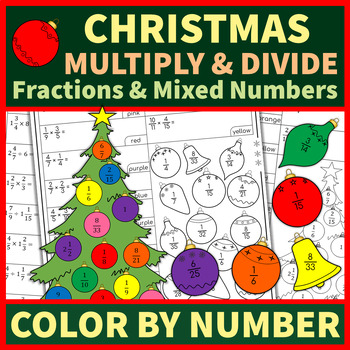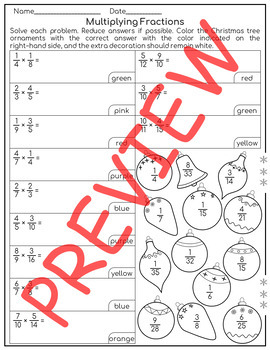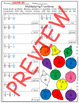# Christmas Worksheets: Multiplication and Division of Fractions and Mixed NumbersSubject
Resource Type
File Type

PDF

(715 KB|4 pages)
Product Rating
Standards
• Product Description
• StandardsNEW

This product contains 2 worksheets:

Multiplication and Division of Fractions

Multiplication and Division of Fractions and Mixed Numbers

A student should solve the problem and color the ball or gift with the correct answer in the color indicated on the right. An «extra» ball /gift should remain white. The answers are included.

Interpret and compute quotients of fractions, and solve word problems involving division of fractions by fractions, e.g., by using visual fraction models and equations to represent the problem. For example, create a story context for (2/3) ÷ (3/4) and use a visual fraction model to show the quotient; use the relationship between multiplication and division to explain that (2/3) ÷ (3/4) = 8/9 because 3/4 of 8/9 is 2/3. (In general, (𝘢/𝘣) ÷ (𝘤/𝘥) = 𝘢𝘥/𝘣𝘤.) How much chocolate will each person get if 3 people share 1/2 lb of chocolate equally? How many 3/4-cup servings are in 2/3 of a cup of yogurt? How wide is a rectangular strip of land with length 3/4 mi and area 1/2 square mi?
Solve real world problems involving multiplication of fractions and mixed numbers, e.g., by using visual fraction models or equations to represent the problem.
Apply and extend previous understandings of multiplication to multiply a fraction or whole number by a fraction.
Total Pages
4 pages
Included
Teaching Duration
N/A
Report this Resource to TpT
Reported resources will be reviewed by our team. Report this resource to let us know if this resource violates TpT’s content guidelines.